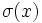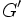# Preserves conjugacy classes for a generating set implies IA

This article gives the statement and possibly, proof, of an implication relation between two automorphism properties. That is, it states that every automorphism satisfying the first automorphism property (i.e., automorphism that preserves conjugacy classes for a generating set) must also satisfy the second automorphism property (i.e., IA-automorphism)
View all automorphism property implications | View all automorphism property non-implications
Get more facts about automorphism that preserves conjugacy classes for a generating set|Get more facts about IA-automorphism

## Statement

Suppose$G$ is a group,$S$ is a generating set for$G$, and$\sigma$ is an automorphism of$G$ such that$\sigma(x)$ is in the conjugacy class of$x$ for all$x \in S$. Then,$\sigma$ is an IA-automorphism of$G$, i.e., the automorphism induced by$\sigma$ on the abelianization of$G$ (the quotient group$G/G'$ of$G$ by its derived subgroup$G' = [G,G]$) is the identity map.

## Proof

Given: A group$G$, a generating set$S$ for$G$, an automorphism$\sigma$ of$G$ such that, for every$x \in S$, there exists$g \in G$ for which$\sigma(x) = gxg^{-1}$.$G'$ is the derived subgroup of$G$ and$\varphi: G \to G/G'$ is the quotient map to the abelianization$G/G'$.

To prove: For every$u \in G$,$\varphi(u) = \varphi(\sigma(u))$

Proof: The proof has two steps:

1. We first show the statement to be proved for all elements of the generating set$S$.
2. We then extend to all of$G$ using the fact that both$\varphi$ and$\varphi \circ \sigma$ are homomorphisms.